• ## Basics of the Laplace Filters

The Laplace filter is a family of highpass filters aimed at edge detection. See; Wikipedia (deutsch).

Variations of the Laplace Filter:

1. The most simple version are the 2x1 Laplace filters.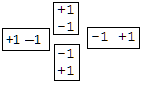This Laplace Filter is a combination of 4 micro-kernels. Each pixel just computes the maximal value of the four differences to its four nearest neighbours. Each step needs 4 subtractions, 4 comparisons to find the maximum and a final multiplication to increase the contrast.
2. The most common version is the 3x3 Laplace filter.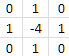The most common version is the 3x3 Laplace filter with a 3x3 kernel of 4 values `0` in the edges, 4 values `1` of the four nearest neighbors surrounding a central value of `-4`.
3. Another variation of 2.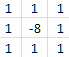This is a 3x3 Laplace filter with a simple 3x3 kernel of 8 values `1` surrounding a central value of `-8`.
4. Most sophisticated version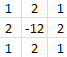A rotation symmetric 3x3 Laplace filter with a 3x3 kernel of 4 values `1` in the edges, 4 values `2` of the four nearest neighbors surrounding a central value of `-12`. The resulting value is finally devided by `4`.

The results of theses 4 variants do not differ much. At first sight they seem to produce identical results.
Version 1 has the advantage that the filter is a composition of four filters, that can be observed separatly,
Declining and ascending edges as well as horizontal edges and vertical edges can be observed as four separated results.
For this reason I recommend the most simple version 1.
All versions have the same border problem.
They cannot filter the outmost rows and columns and any Laplace-filtered image has to leave these borders black.

# C#-Code of the `LaplaceFilter`:

```//C#-Code of the LaplaceFilter:
```
• ## Five 2x1 Laplace Filters Of Real Images

```/* This function "diff" is called 12 times by "LaplaceFilter". ***************/
/* The global value "ContrastFactor" is set by a slider of the GUI ***********/
private int diff( int d ) //enhance difference and clip the result to 0..255
{ if ( d <= 0 ) return 0; //clip negative values to 0
return Convert.ToInt32(Math.Min(255f,  ContrastFactor*d));
}
private void LaplaceFilter( DoWorkEventArgs e )
{ int R, G, B, Rmax, Gmax, Bmax, pointer;
for ( int y=0; y < ySize-1; y++ )
pointer = y*xSize; //points now to the first pixel of row y
for ( int x=0; x < xSize-1; x++, pointer++ )
{ R = diff( R0[y,x+1] - R0[y,x] ); Rmax = R;
G = diff( G0[y,x+1] - G0[y,x] ); Gmax = G;
B = diff( B0[y,x+1] - B0[y,x] ); Bmax = B;
unchecked { imRML.Pixels[pointer] = (Int32)0xff000000 | R << 16 | G << 8 | B; }
R = diff( R0[y,x] - R0[y,x+1] ); Rmax = Math.Max(R, Rmax);
G = diff( G0[y,x] - G0[y,x+1] ); Gmax = Math.Max(G, Gmax);
B = diff( B0[y,x] - B0[y,x+1] ); Bmax = Math.Max(B, Bmax);
unchecked { imLMR.Pixels[pointer] = (Int32)0xff000000 | R << 16 | G << 8 | B; }
R = diff( R0[y+1,x] - R0[y,x] ); Rmax = Math.Max(R, Rmax);
G = diff( G0[y+1,x] - G0[y,x] ); Gmax = Math.Max(G, Gmax);
B = diff( B0[y+1,x] - B0[y,x] ); Bmax = Math.Max(B, Bmax);
unchecked { imLMU.Pixels[pointer] = (Int32)0xff000000 | R << 16 | G << 8 | B; }
R = diff( R0[y,x] - R0[y+1,x] ); Rmax = Math.Max(R, Rmax);
G = diff( G0[y,x] - G0[y+1,x] ); Gmax = Math.Max(G, Gmax);
B = diff( B0[y,x] - B0[y+1,x] ); Bmax = Math.Max(B, Bmax);
unchecked { imUML.Pixels[pointer] = (Int32)0xff000000 | R << 16 | G << 8 | B; }
unchecked { imAll.Pixels[pointer] = (Int32)0xff000000 | Rmax << 16 | Gmax << 8 | Bmax; }
}
}
}
```
• ## Sobel Filter Of Real Images

The Sobel Filter is the most prominent of the so called gradient filters such as Sobel, Prewitt, Roberts, Canny filters.
A gradient is a vector of the directional change in the intensity.
It has two values: direction `θ` (=theta) and length `G`.
Both `θ` and `G` can be computed from a horizontal and a vertical gray value difference.
Therefore any gradient filter needs two filtering operations with two different kernels: `kx` and `ky`.
`kx` for horizontal and `ky` for vertical changes resulting in two independent (positive or negative) differences `Gx` and `Gx`.
In a third step `θ` and `G` can be computed from `Gx` and `Gx`.
In practice nobody is interested in `θ`, the output gray value is simply computed from `G`.
The Sobel filter is defined by:

 `kx` ` = `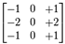`kx` ` = `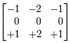`G` ` = `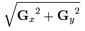`output` ` = ` `(Byte)(ContrastFactor * G);`
```private void SobelFilter( DoWorkEventArgs e )
{ int Rh, Gh, Bh, Rv, Gv, Bv,  R, G, B, pointer;
int Rul, Gul, Bul; //upper left              3x3 Kernel scheme:
int Rum, Gum, Bum; //upper mid                  Xul Xum Xur
int Rur, Gur, Bur; //upper right                Xml Xmm Xmr
int Rml, Gml, Bml; //mid   left                 Xll Xlm Xlr
int Rmm, Gmm, Bmm; //mid   mid
int Rmr, Gmr, Bmr; //mid   right
int Rll, Gll, Bll; //lower left
int Rlm, Glm, Blm; //lower mid
int Rlr, Glr, Blr; //lower right
ContrastFactor /= 4; //The Sobel result is up to 4*255 (white minus black).
for ( int y=1; y < ySize-1; y++ )
pointer = y*xSize; //points now to the first pixel of row y
for ( int x=1; x < xSize-1; x++, pointer++ )
{ //rename upper kernel row
Rul = R0[y-1,x-1]; Gul = G0[y-1,x-1]; Bul = B0[y-1,x-1];
Rum = R0[y-1,x  ]; Gum = G0[y-1,x  ]; Bum = B0[y-1,x  ];
Rur = R0[y-1,x+1]; Gur = G0[y-1,x+1]; Bur = B0[y-1,x+1];
//rename mid kernel row
Rml = R0[y  ,x-1]; Gml = G0[y  ,x-1]; Bml = B0[y  ,x-1];
Rmm = R0[y  ,x  ]; Gmm = G0[y  ,x  ]; Bmm = B0[y  ,x  ]; //just for
Rmr = R0[y  ,x+1]; Gmr = G0[y  ,x+1]; Bmr = B0[y  ,x+1];
//rename lower kernel row
Rll = R0[y+1,x-1]; Gll = G0[y+1,x-1]; Bll = B0[y+1,x-1];
Rlm = R0[y+1,x  ]; Glm = G0[y+1,x  ]; Blm = B0[y+1,x  ];
Rlr = R0[y+1,x+1]; Glr = G0[y+1,x+1]; Blr = B0[y+1,x+1];
//horizontal Sobel
Rh = -Rul + Rur - 2*Rml + 2*Rmr - Rll + Rlr;
Gh = -Gul + Gur - 2*Gml + 2*Gmr - Gll + Glr;
Bh = -Bul + Bur - 2*Bml + 2*Bmr - Bll + Blr;
//vertical Sobel
Rv = -Rul + Rll - 2*Rum + 2*Rlm - Rur + Rlr;
Gv = -Gul + Gll - 2*Gum + 2*Glm - Gur + Glr;
Bv = -Bul + Bll - 2*Bum + 2*Blm - Bur + Blr;
R = Math.Min(255, Convert.ToInt32(ContrastFactor * Math.Sqrt(Rh*Rh + Rv*Rv)));
G = Math.Min(255, Convert.ToInt32(ContrastFactor * Math.Sqrt(Gh*Gh + Gv*Gv)));
B = Math.Min(255, Convert.ToInt32(ContrastFactor * Math.Sqrt(Bh*Bh + Bv*Bv)));
//uplod a 32-bit ARGB-pixel
unchecked { imgOut.Pixels[pointer] = (Int32)0xff000000 | R << 16 | G << 8 | B; }
}
}
}```

******************************************************************************************
Q: What is a Highpass filter ? Purpose ? Kernels ?
A: A HP filter passes high frequncies and blocks low frequencies.
It reduces all homogenous areas to black areas and preserves just those parts of the image where gray values abruptly change.
It is used for edge detection and sharpening.
HP filters have at least two kernels (one for horizontal and another for vertical edges).
These kernels always contain (among positive or null values) negative values.
******************************************************************************************
Q: What are Laplace filters ? Simplest Laplace filter ?
A: A family of lightweight highpass filters performing simple horizontal and vertical subtractions.
The simplest Laplace filter subtracts every pixel from its 4 direct neightbors and takes the maximum.
******************************************************************************************
Q: What is a gradient filter ?
A: It combines the horizontal and the vertical differences `Gx` and `Gy` to one (non-negative) value `G = Math.Sqrt(Gx*Gx + Gy*Gy)`.
******************************************************************************************
Q: The two kernels of the Sobel filter ?
A:******************************************************************************************